# 单智能体强化学习算法

## Rainbow：Combining Improvements in Deep Reinforcement Learning

Posted by MY on August 5, 2020

## 一、问题

Rainbow主要探究了如下几种算法在不同方面对DQN的改进的作用，并进行了这些改进的组合。

## 二、解法

### 2.1 DQN

#### 2.1.1 算法简介

• 数字列表项目观察序列中存在相关性
• 对当前Q的小步更新都可能显著影响策略，而今使更新后的策略采集的数据分布和更新前的不一致，进而改变Q值与目标值之间的相关性

• Replay Buffer：强化学习训练时会将智能体的经验根据时间顺序连续保存到经验回放池中$(s,a,r,s’)$，会造成相邻两个样本之间存在相关性，解决思路是利用随机均匀采样经验池的中样本数据供智能体训练
• Fixed Q-Target：DQN使用定期迭代更新的方式更新目标值计算中的拟合网络参数，从而减少了与目标值之间的相关性，如果一直更新，会由于目标值一直改变不”稳定”而造成训练发散

DQN迭代更新的损失函数为：

$\operatorname{Loss}_{i}\left(\theta_{i}\right)=\mathbb{E}_{\left(s, a, r, s^{\prime}\right) \sim U(D)}\left[\left(r+\gamma \max _{a^{\prime}} Q\left(s^{\prime}, a^{\prime} ; \theta_{i}^{-}\right)-Q\left(s, a ; \theta_{i}\right)\right)^{2}\right]$

• $\gamma$是折扣因子，用来决定目标值对于当前值的影响程度
• $\theta_{i}$代表第i次迭代过程的神经网络参数
• $\theta_{i}^{-}$代表目标值函数的神经网络参数
• 目标值函数的网络参数是每隔$C$次训练才更新，$C$次训练期间保持目标值函数的网络参数固定不变

#### 2.1.2 代码实现

• collections.deque: 底层实现是使用的双向链表，插入时间复杂度是O(1)，但是查询的时间复杂度是O(n)，会严重增加训练过程的时间
• list：列表底层是数组，存储的是指向不同或者相同大小物体的指针，可以动态增加数据和支持随机访问，比使用deque队列的方式要快
• numpy.ndarray：访问最快的数据结构，底层是存储的具有相同固定大小的同质数据，可以根据内存的访问局部性原理加快读取速度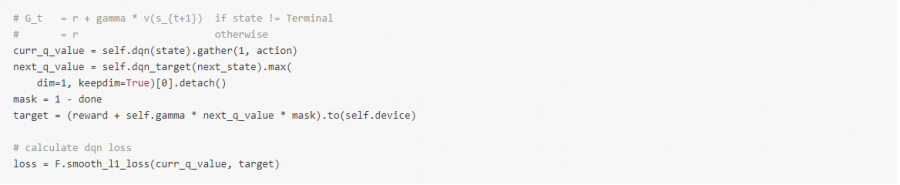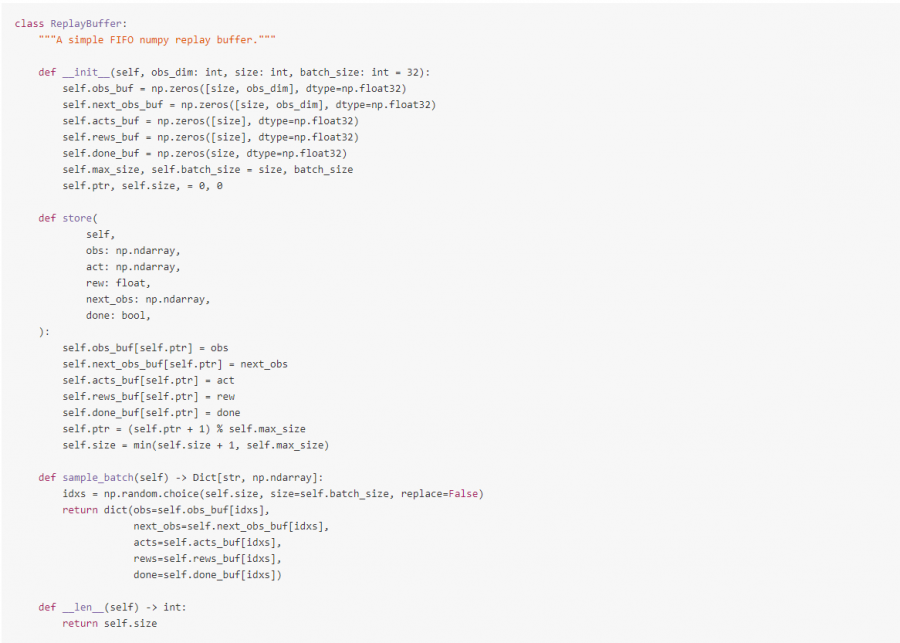### 2.2 Double DQN

#### 2.2.1 算法简介

DQN的目标函数：

$Y_{t}^{Q}=R_{t+1}+\gamma \max _{a} Q\left(S_{t+1}, \arg \max _{a} Q\left(S_{t+1}, a ; \theta_{t}\right) ; \theta_{t}\right)$

DDQN的目标函数为：

$Y_{t}^{\text {DoubleQ }}=R_{t+1}+\gamma \max _{a} Q\left(S_{t+1}, \text { arg } \max _{a} Q\left(S_{t+1}, a ; \theta_{t}\right) ; \theta_{t} \prime\right)$

#### 2.2.2 代码实现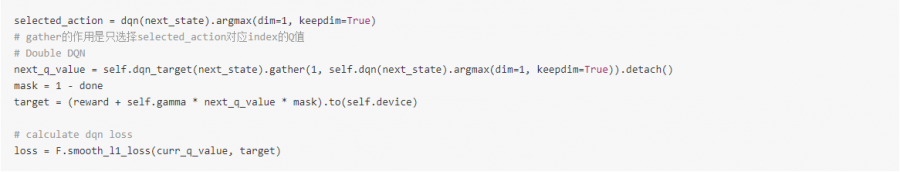### 2.3 Prioritized Experience Replay (PER)

#### 2.3.1 算法简介

• 存储哪些动作序列
• 采样哪些动作序列

PER要解决的是第二个问题，即如何最有效地选择经验回放池中的样本数据进行学习，其核心思想是衡量每个动作序列样本的重要性程度。一种可行的方法是利用样本的TD-error来表示，因为这表明了这次样本的“惊喜”大小。该算法会存储经验回放池中每个样本的最后的TD-error值，然后根据最大绝对TD-error进行采样。Q-learning会利用采样到的样本进行训练和根据TD-error更新权重。当新的转换无法计算TD-error时，会将其误差设置为最大，从而保证所有的样本至少被采样一次。

• Greedy TD-Error Prioritization: 最大的缺点是会聚焦于一小部分经验子集，当使用函数逼近的方法时，误差减小的慢，意味着一开始高误差的样本会被频繁重复地采样，这样的欠多样性样本使得训练的智能体很容易就过拟合
• Stochastic Prioritization：介于纯Greedy Prioritization和均匀随机采样之间:$P(i)=\frac{p_{i}^{\alpha}}{\sum_{k} p_{k}^{\alpha}}$。其中$p_{i}$代表样本$i$的优先级大小，指数$\alpha$代表使用优先级的影响程度，当$\alpha$为0时，代表均匀随机采样。在实际中，会添加一个正整数偏置常量$\epsilon$，$p_i=|\sigma_i| + \epsilon$，保证可以对所有的样本进行采样。

$w_{i}=\left(\frac{1}{N} \cdot \frac{1}{P(i)}\right)^{\beta}$

#### 2.3.2 代码实现

PrioritizedReplayBuffer的实现利用了Segment Tree数据结构，它在保存经验样本和采样样本的同时也负责管理样本的Priorities，是非常高效的实现方式。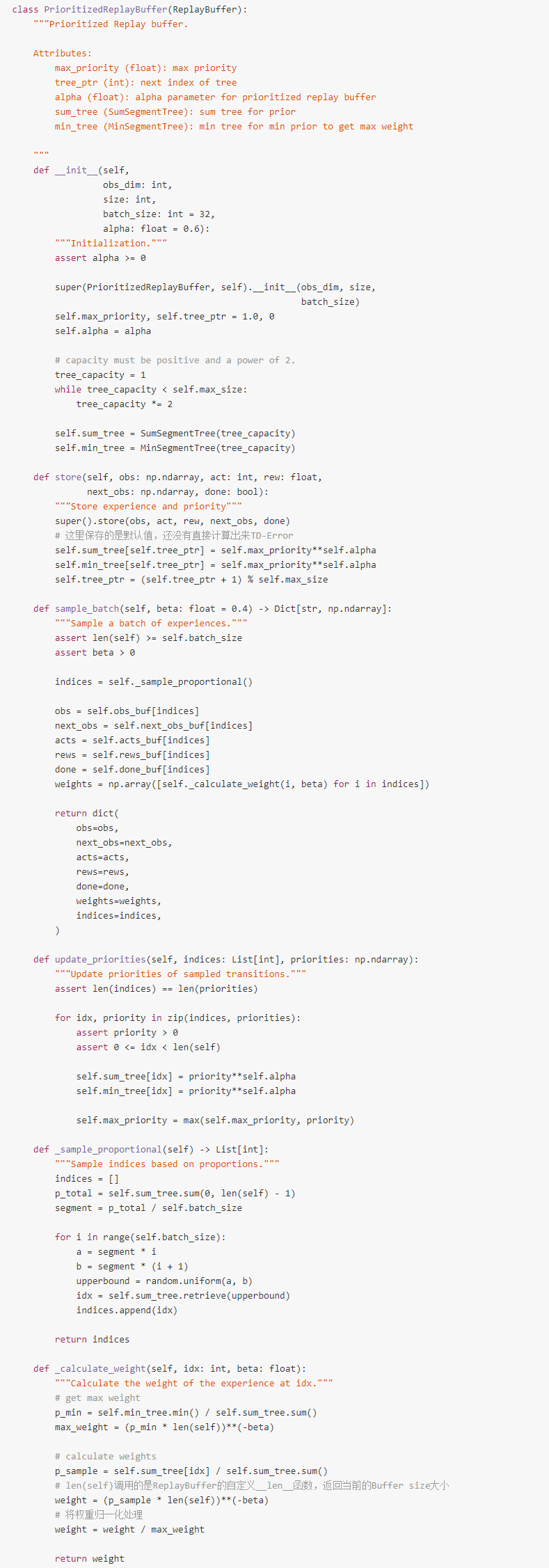Huber loss也就是通常所说的SmoothL1 loss，其对于异常点的敏感性不如MSE，在某些情况下防止了梯度爆炸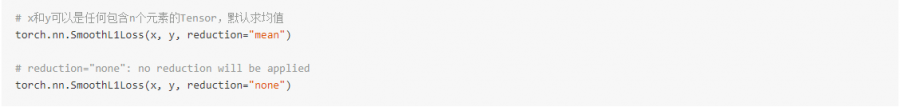### 2.4 Dueling Network

#### 2.4.1 算法简介

$A^{\pi}(s, a)=Q^{\pi}(s, a)-V^{\pi}(s)$

$Q(s, a ; \theta, \alpha, \beta)=V(s ; \theta, \beta)+A(s, a ; \theta, \alpha)$

$Q(s, a ; \theta, \alpha, \beta)=V(s ; \theta, \beta)+\left(A(s, a ; \theta, \alpha)-\max _{a^{\prime} \in[.4]} A(s, a \prime ; \theta, \alpha)\right)$

$Q(s, a ; \theta, \alpha, \beta)=V(s ; \theta, \beta)+\left(A(s, a ; \theta, \alpha)-\frac{1}{\|\mathcal{A}\|} \sum_{a^{\prime}} A(s, a \prime ; \theta, \alpha)\right)$

#### 2.4.2 代码实现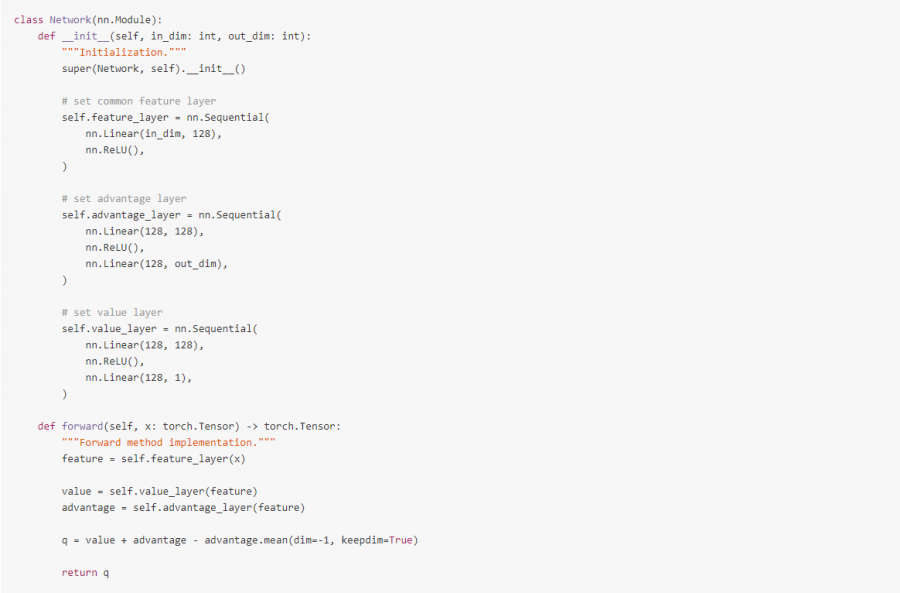### 2.5 Noisy Networks for Exploration

#### 2.5.1 算法简介

NoisyNet是一种探索方法，可以通过学习网络权重的扰动来促进策略的探索，主要认为对权重向量的微小更改会造成多个时间步中引发一致且可能非常复杂的状态相关的策略更改。

$y=wx+b$

$y=\left(\mu^{w}+\sigma^{w} \odot \epsilon^{w}\right) x+\mu^{b}+\sigma^{b} \odot \epsilon^{b}$

• Independent Gaussian Noise: 每一个添加给权重和偏置的随机噪声都是从单位高斯分布中采样和独立的，对于每一个线性神经元来说，将会有$pq+q$个噪声变量
• Factorised Gaussian Noise: 该方法对于运算来说非常高效。它通过生成2个随机高斯噪声向量$(p,q)$的外积来产生$pq+q$个随机噪声：$\begin{array}{c} \epsilon_{i, j}^{w}=f\left(\epsilon_{i}\right) f\left(\epsilon_{j}\right) \\ \epsilon_{j}^{b}=f\left(\epsilon_{i}\right) \\ f(x)=\operatorname{sgn}(x) \sqrt{|x|} \end{array}$

#### 2.5.2 代码实现

NoisyNet可以看做是$\epsilon$-greedy的一种，因此与$\epsilon$有关的代码都可以省去。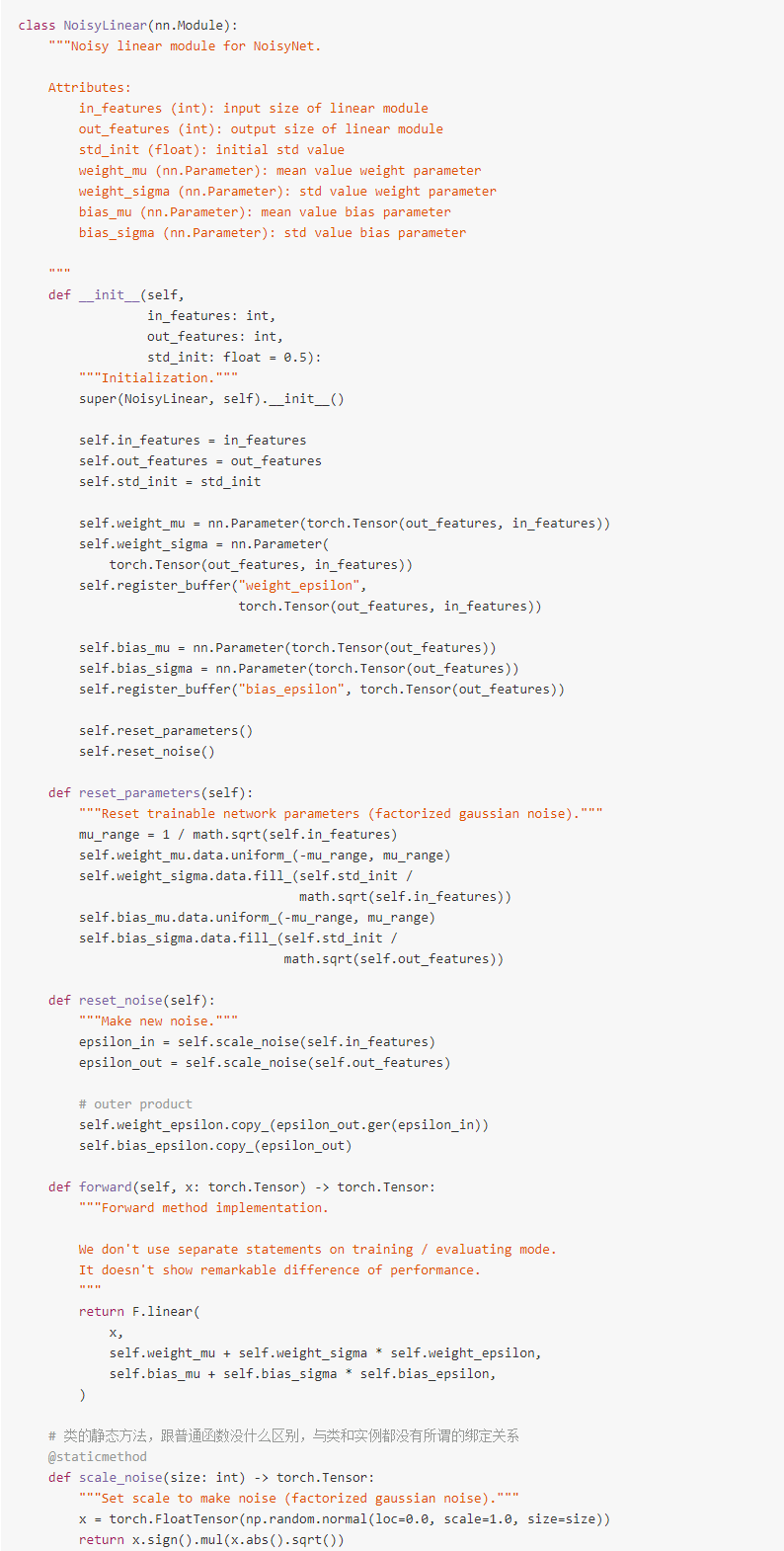### 2.6 Categorical DQN

#### 2.6.1 算法简介

$d_{t^{\prime}}=\left(R_{t+1}+\gamma_{t+1} z, p_{h} a t \theta\left(S_{t+1}, \hat{a}_{t+1}^{*}\right)\right)$

$D_{K L}\left(\phi_{z} d_{t^{\prime}} | d_{t}\right)$

#### 2.6.2 代码实现

$\begin{array}{c} z_{i}=V_{\min }+i \Delta z: 0 \leq i<N, \Delta z=\frac{V_{\max }-V_{\min }}{N-1} \\ Q\left(s_{t}, a_{t}\right)=\sum_{i} z_{i} p_{i}\left(s_{t}, a_{t}\right) \end{array}$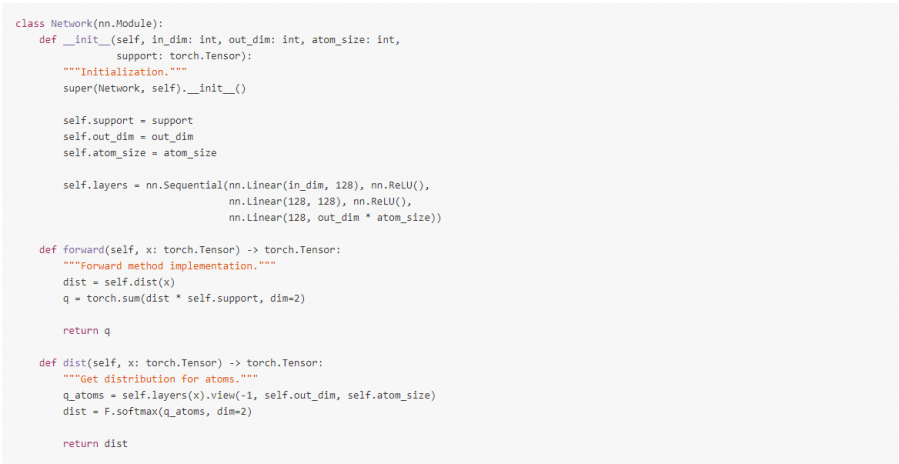### 2.7 N-step Learning

#### 2.7.1 算法简介

Q-learning会利用一个单一的奖惩值和下一个状态下最优动作的Q值来进行自举(Bootstrap)，该方法只能利用下一个时刻的信息，改进思路是向前多看几步，利用接下来的N步信息，这就是在状态$S_t$下的Truncated N-Step Return，定义为：$R_{t}^{(n)}=\sum_{k=0}^{n-1} \gamma_{t}^{(k)} R_{t+k+1}$ DQN的变体N-Step Learning的目标是通过最小化下面的损失函数：

$\left(R_{t}^{(n)}+\gamma_{t}^{(n)} \max _{a^{\prime}} q_{\theta}^{-}\left(S_{t+n}, a^{\prime}\right)-q_{\theta}\left(S_{t}, A_{t}\right)\right)^{2}$

#### 2.7.2 代码实现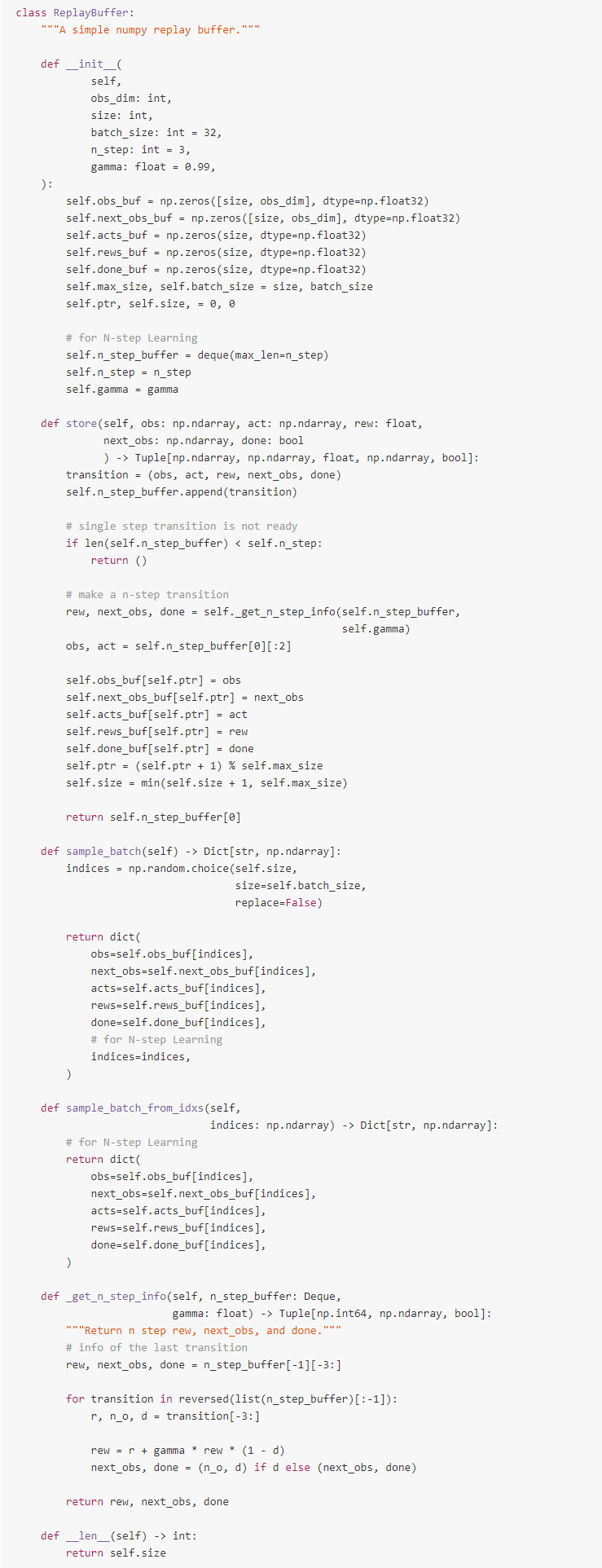## 三、实验内容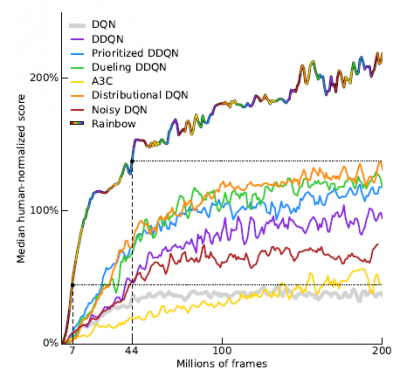## 五、优点

##### Share